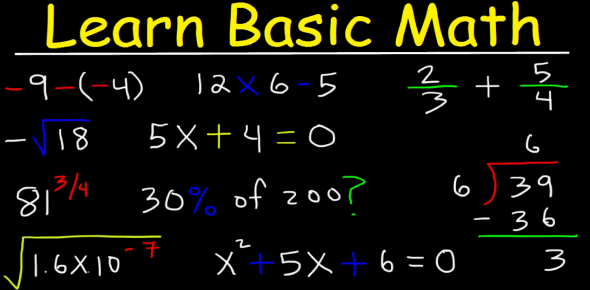# General Arithmetic Quiz

20 Questions | Total Attempts: 243Settings.

• 1.
1)Compiler generates ___ file.
• A.

Executable code

• B.

Object code

• C.

Assembly code

• D.

None of the above

• 2.
Identify the C compiler of UNIX.
• A.

Gcc

• B.

Cc

• C.

Borland

• D.

Vc++

• 3.
A macro can execute faster than a function.
• A.

True

• B.

False

• 4.
Choose the correct unary operators in C – a) !, b) ~, c) ^&, d) ++
• A.

A,b,d

• B.

A,b,c

• C.

B,c,d

• D.

C,d,a

• 5.
Which library functions help users to dynamically allocate memory?
• A.

Memalloc()and alloc()

• B.

Malloc() and memalloc()

• C.

Malloc() and calloc()

• D.

Memalloc() and calloc()

• 6.
What is the size of ‘int’?
• A.

2

• B.

4

• C.

8

• D.

Compiler dependent

• 7.
C is the successor of ___ programming language.
• A.

C++

• B.

B++

• C.

B

• D.

Mini c

• 8.
The binary equivalent of 50 is,
• A.

110010

• B.

1010110

• C.

101

• D.

101.011.00.

• 9.
In the standard library of C programming language, which of the following header file is designed for basic mathematical operations?
• A.

Math.h

• B.

Conio.h

• C.

Dos.h

• D.

Stdio.h

• 10.
Which of the following operator can be used to access value at address stored in a pointer variable?
• A.

*

• B.

#

• C.

&&

• D.

@

• 11.
In C, what is the correct hierarchy of arithmetic operations?
• A.

*/ + -

• B.

* +- /

• C.

/ *+ -

• D.

+ - / *

• 12.
What is the output of the following program?#include<stdio.h>main(){       fprintf(stdout,"Hello, World!");}
• A.

Hello, World!

• B.

No output

• C.

Compiler error

• D.

Runtime error

• 13.
Which of the following is a logical AND operator?
• A.

!

• B.

&&

• C.

| |

• D.

&

• 14.
Which statement can print \n on the screen?
• A.

Printf("\\n");

• B.

Printf("n\");

• C.

Printf("n");

• D.

Printf('\n');

• 15.
How many types of polymorphisms is supported by C++?
• A.

4

• B.

2

• C.

0

• D.

1

• 16.
Which of the following is an abstract data type?
• A.

Class

• B.

Int

• C.

String

• D.

Double

• 17.
Which of the following approach is adopted in  C++?
• A.

Top-down

• B.

Bottom-up

• C.

Right-left

• D.

None

• 18.
Which of the following  cannot be used as with the keyword virtual?
• A.

Class

• B.

Member functions

• C.

Constructor

• D.

Destructor

• 19.
Which of the following is not a type of inheritance?
• A.

Multilevel

• B.

Multiple

• C.

Distributive

• D.

Hierarchial

• 20.
Which of the following operators cannot be overloaded?
• A.

[ ]

• B.

->

• C.

?:

• D.

*

• 21.
Which of the following statements is correct in C++?
• A.

Classes cannot have data as protected members.

• B.

Structures can have functions as members.

• C.

Class members are public by default.

• D.

Structure members are private by default.

• 22.
Which of the following statement is correct?
• A.

Class is an instance of object.

• B.

Object is an instance of a class.

• C.

Class is an instance of data type.

• D.

Object is an instance of data type.

• 23.
Which of the following functions are performed by a constructor?
• A.

Construct a new class

• B.

Construct a new object

• C.

Construct a new function

• D.

Initialize objects

• 24.
Which of the following problem causes an exception?
• A.

Missing semicolon in statement in main()

• B.

A problem in calling function.

• C.

A syntax error

• D.

A run-time error

• 25.
Can you analyse what is the probability of meeting someone in your lifetime who is having an above average number of arms?
• A.

Impossible

• B.

Unlikely

• C.

Fifty Fifty

• D.

Fairly Likely

• E.

Certain

Related Topics